Homework Help Question & Answers

# The acceleration of particle is given as a function of velocity, a(v), find the position as...

The acceleration of particle is given as a function of velocity, a(v), find the position as a function of velocity, v(s)

#### Homework Answers

Answer #1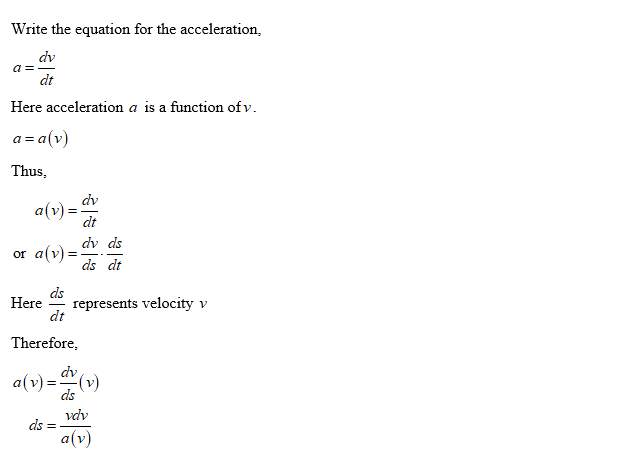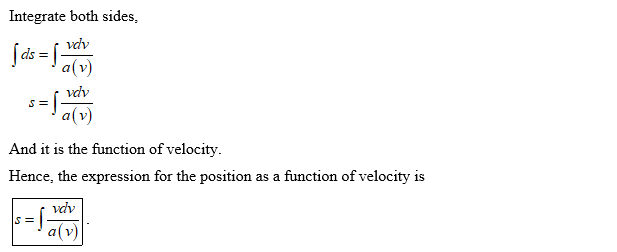Know the answer?
Your Answer:

#### Post as a guest

Your Name:

What's your source?

#### Earn Coin

Coins can be redeemed for fabulous gifts.

Not the answer you're looking for? Ask your own homework help question. Our experts will answer your question WITHIN MINUTES for Free.
Similar Homework Help Questions
• ### The acceleration of particle is given as a function of velocity afy ind the position asa...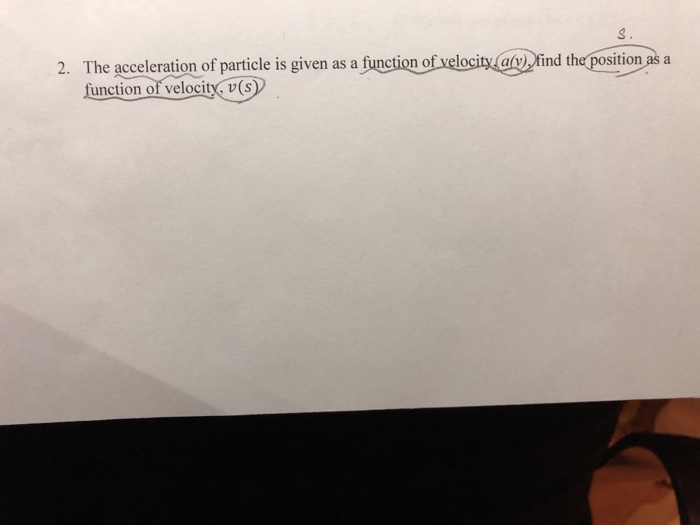The acceleration of particle is given as a function of velocity afy ind the position asa function of velocity, v(s 2.

• ### Find the velocity and position vectors of a particle that has the given acceleration and the given initial velocity and position

Find the velocity and position vectors of a particle that has the given acceleration and the given initial velocity and position. a(t)= 3i + 2j, v(0)= k, r(0)=ifind r(t).

• ### Find the position function x(t) of a moving particle with the given acceleration a(t), initial position...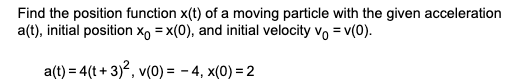Find the position function x(t) of a moving particle with the given acceleration a(t), initial position Xo = x(0), and initial velocity vo = v(O). a(t) = 4(t+3)2, v(0) = - 4, x(0) = 2

• ### The acceleration function (in m/s2) and the initial velocity v(o) are given for a particle moving...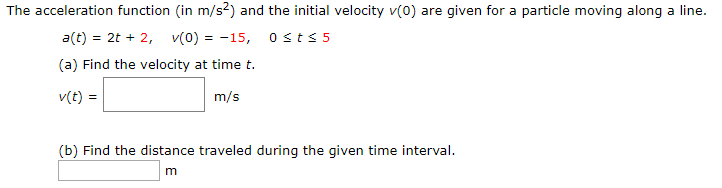The acceleration function (in m/s2) and the initial velocity v(o) are given for a particle moving along a line. a(t) = 2t + 2, VO) = -15, Osts 5 (a) Find the velocity at time t. v(t) = m/s (b) Find the distance traveled during the given time interval.

• ### The acceleration function (in m/s2) and the initial velocity are given for a particle moving along...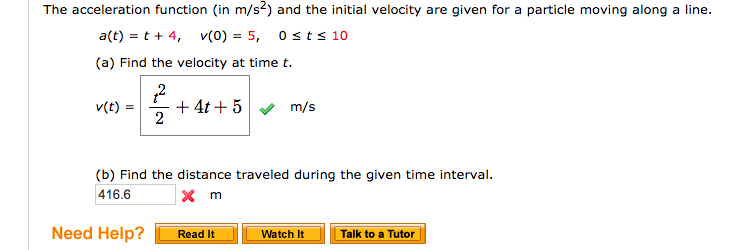The acceleration function (in m/s2) and the initial velocity are given for a particle moving along a line. a(t) t 4 5, 0sts 10 v(0) (a) Find the velocity at time t. 2 4t5 2 v(t) m/s (b) Find the distance traveled during the given time interval. 416.6 Need Help? Talk to a Tutor Read It Watch It The acceleration function (in m/s2) and the initial velocity are given for a particle moving along a line. a(t) t 4 5,...

• ### Find the position vector for a particle with acceleration, initial velocity, and initial position given below. a(t...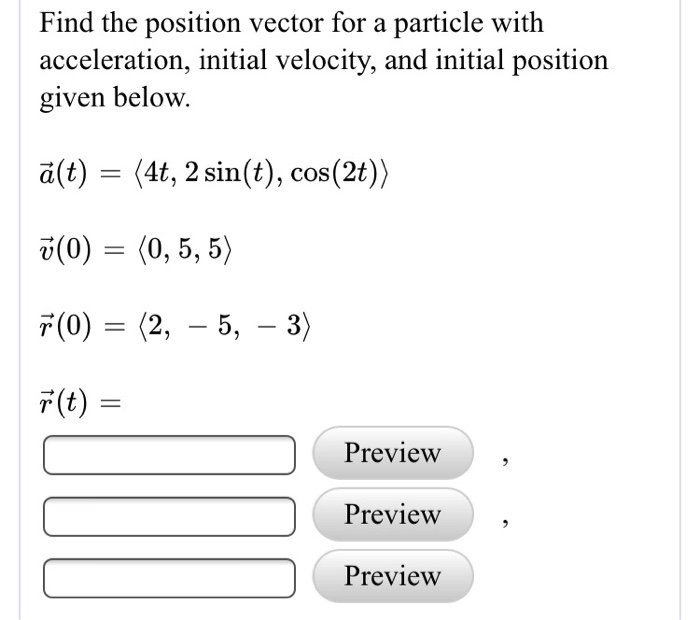Find the position vector for a particle with acceleration, initial velocity, and initial position given below. a(t) (4t, 2 sin(t), cos(2t)) 5(0) (0, 5,5) r(t) Preview Preview Preview The position of an object at time t is given by the parametric equations Find the horizontal velocity, the vertical velocity, and the speed at the moment wheret - 4. Do not worry about units in this problem. Horizontal Velocity - Preview Vertical Velocity- Preview Preview peed- Find the position vector for...

• ### * A particle is moving with acceleration function a(t) = 21-1, find the position of the...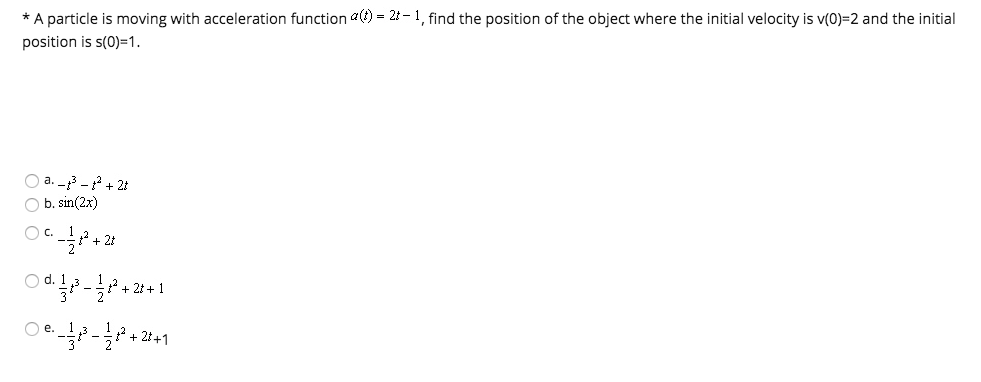* A particle is moving with acceleration function a(t) = 21-1, find the position of the object where the initial velocity is v(O)=2 and the initial position is s(0)=1. a. -3 -2 +21 b.sin(2x) OC 12 +2 Od. - *+21+1 Oe 12-*+2+1

• ### Suppose the initial velocity of a particle is given by v(O)=(-1,0,0) and the acceleration is given...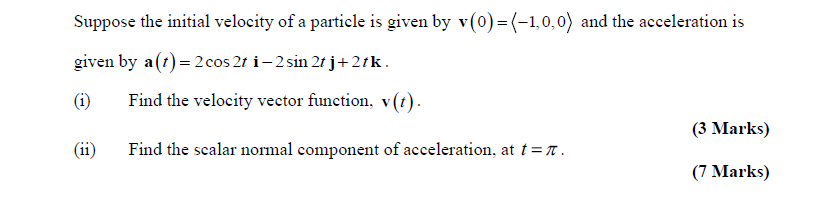Suppose the initial velocity of a particle is given by v(O)=(-1,0,0) and the acceleration is given by a(t)=2cos 2t i-2 sin 2t j+2tk. (1) Find the velocity vector function, v(t). (3 Marks) Find the scalar normal component of acceleration, at trī. (7 Marks)

• ### 9. A particle moves along the x-axis so that its velocity v at time t, for0 sts 5, is given by v(t) In(t2-3t +3). The particle is at position x 8 at time t 0. a) Find the acceleration of the part...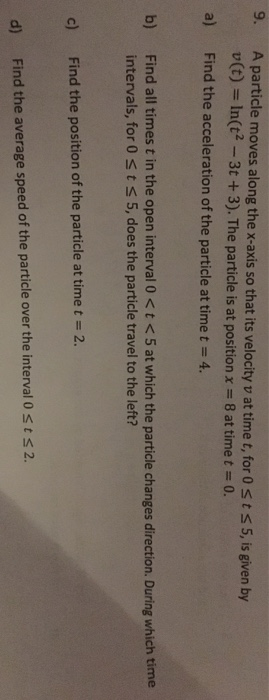9. A particle moves along the x-axis so that its velocity v at time t, for0 sts 5, is given by v(t) In(t2-3t +3). The particle is at position x 8 at time t 0. a) Find the acceleration of the particle at time t 4. b) Find all times t in the open interval 0<t <5 at which the particle changes direction. During which time intervals, for 0st s 5, does the particle travel to the left? c) Find...

• ### 9. [10 pts] Find the original position and velocity functions for a particle if it's acceleration...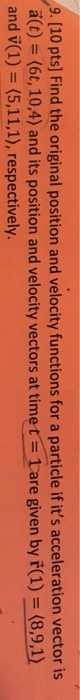9. [10 pts] Find the original position and velocity functions for a particle if it's acceleration vector is a(t) (6t, 10,4) and its position and velocity vectors at timet 1are given by ř(1) (8,9,1) and v(1) (5,11,1), respectively.